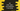# C# program to find the index of an element in an array## C# program to find the index of an element in an array:

To find the index of an element in an array in C#, it provides one method called FindIndex which is defined in the Array class. We can use this method to find the index of one element in a given array.

In an array in C#, the index starts at 0. So, the first element is at the 0th index, the second is at the 1st, etc.

In this post, we will learn how to use this method with different examples. It also has three overloads that we will check in this post.

### Definition of FindIndex:

FindIndex method has three overloads. These are defined as below:

#### 1

``FindIndex<T>(T[], Predicate<T>)``

It takes one array and one Predicate. It searches in the array and uses the Predicate to find the first element that matches this Predicate. If it finds any item, it returns the index of the first.

#### 2

``FindIndex<T>(T[], Int32, Predicate<T>)``

This is similar to the above one. The only difference is that it starts the search from the index we are providing in the second parameter. The search ends at the end of the array.

#### 3

``FindIndex<T>(T[], Int32, Int32, Predicate<T>)``

It starts the searches as defined in the second argument and searches for the third argument number of elements.

### Return value of FindIndex:

FindIndex returns the index of the element if it is found. Else it returns -1

### Exception by FindIndex:

It throws ArgumentNullException if any of the argument is null. If the array is null or if the predicate is null, it will throw this exception.

### Example program:

Let’s take a look at the below program:

``````using System;

public class Program
{
public static void Main()
{
int[] givenArr = new int[] { 1, 2, 3, 4, 5, 6, 7, 8, 9, 10, 1, 2, 3, 4, 5 };
Console.WriteLine(Array.FindIndex(givenArr, item => item == 1));
Console.WriteLine(Array.FindIndex(givenArr, 2, item => item == 1));
Console.WriteLine(Array.FindIndex(givenArr, 2, 8, item => item == 1));
}
}``````

Here,

• givenArr is the given array with integers.
• The first WriteLine is finding the index of 1 in that array. We have two 1 in the array. But, it will return the index of the first 1 from the array.
• The second WriteLine is returning the index of the second 1. Because the search starts from index 2 to the end.
• The third WriteLine returns -1. Because it is searching for 1 from index 2 and checks for 8 numbers.

If you run this program, it will print the below output:

``````0
10
-1``````

### Passing a function as a predicate:

We can pass one function as the Predicate. Using a function has many advantages like we can write more complex logics inside this function. Let’s rewrite the above program using a function as the Predicate:

``````using System;

public class Program
{
static bool isItOne(int item)
{
return item == 1;
}

public static void Main()

{
int[] givenArr = new int[] { 1, 2, 3, 4, 5, 6, 7, 8, 9, 10, 1, 2, 3, 4, 5 };

Console.WriteLine(Array.FindIndex(givenArr, isItOne));

Console.WriteLine(Array.FindIndex(givenArr, 2, isItOne));

Console.WriteLine(Array.FindIndex(givenArr, 2, 8, isItOne));

}

}``````

It will print the same output. The advantage of this method is that we can write more complex logic in the function and we don’t have to rewrite the same code for different FindIndex methods.